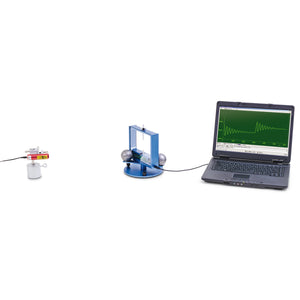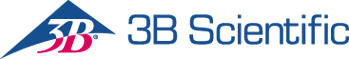3B Scientific

# 3b Scientific Experiment: Gravitational Constant, Basic Equipment

Regular price \$2,500.00 \$0.00 Unit price per

# Experiment: Gravitational Constant, Basic Equipment

Objective: Measure the gravitational force and determine the gravitational constant usual a Cavendish torsion balance

The central component of a Cavendish torsion balance is a sensitive torsional pendulum with a pair of small lead spheres attached to it. Two larger lead spheres are then placed near these two small balls in order to attract them. The position of the large spheres thus determines the equilibrium position of the torsional pendulum. If the two large spheres are then moved to a second position which is symmetrical with the first with respect to the two small balls, the torsional pendulum will adopt a new equilibrium position after a short period of settling. By measuring the geometry of the set-up in both positions, it is possible to determine the gravitational constant. The decisive factor in this is the equilibrium between the gravitational force and the restoring torque of the torsional pendulum. Measurements are made of the oscillation of the torsional pendulum using a capacitive differential sensor, which suppresses noise and vibrational components of the signal to a large extent. The tungsten wire from which the pendulum is made is chosen to be so thin that the period of oscillation is of the order of a few minutes, meaning that several oscillations about the equilibrium position may be observed in the space of an hour.